# Tree Tutorial 2: More Propositional Tree Rules

Logical System

### Skills to be acquired in this tutorial:

To become familiar with the rules for propositional truth trees.

### Tutorial:

#### Truth Tree Rules

Trees are going to be used to 'picture' the truth conditions or requirements for a formula (then, as the technique is developed, for several formulas at once).

Any easy way to remember the rules is to regard them as having been built from the truth tables.

The truth table for 'and' is

Conjunction

 F G (F&G) True True True True False False False True False False False False

For a conjunction to be true, each of its immediate subformulas have to be true. A tree can be extended from a conjunction, and it is extended by adding each of the immediate subformulas to all the branches that the conjunct belongs to. Thus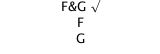The truth table for 'or' is

Disjunction

 F G (F∨G) True True True True False True False True True False False False

For a disjunction to be true, either of its immediate subformulas have to be true. A tree can be extended from a disjunction, and it is extended by adding two sub-branches, one containing one of the immediate subformulas and the other containing the other immediate subformula (to all the branches that the disjunct belongs to). Thus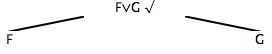The truth table for 'conditional' is

Conditional

 F G (F→G) True True True True False False False True True False False True

For a conditional to be true, either of its antecedent subformula has to be false or its consequent subformula has to be true. A tree can be extended from a conditional, and it is extended by adding two sub-branches, one containing the negation of the antecedent and the other containing the consequent (to all the branches that the conditional belongs to). Thus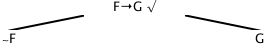The truth table for 'biconditional' is

Biconditional

 F G (F ≡ G) True True True True False False False True False False False True

For a biconditional to be true, either both of its subformula have to be true or both of its subformulas have to be false. A tree can be extended from a biconditional, and it is extended by adding two sub-branches, one containing both the subformulas and the other containing the negations of both the subformulas (to all the branches that the biconditional belongs to). Thus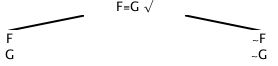You might think we are finished, but we are not!

We need to be able to draw trees for all sentential logic formulas, we need to be able to picture the truth conditions for any formula. To do that we need tree extension rules for the cases when the connective occur in combination with negation.

Here they are

Double Negation

 F ~~F True True False False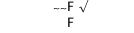Negated Conjunction

 F G ~F&G) True True False True False True False True True False False True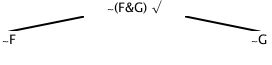Negated Disjunction

 F G ~(F∨G) True True False True False False False True False False False TrueNegated Conditional

 F G ~(F→G) True True False True False True False True False False False FalseNegated Biconditional

 F G ~(F ≡ G) True True False True False True False True True False False False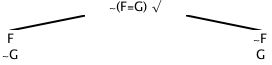#### Example

Here is an example of a larger tree (in all its glory)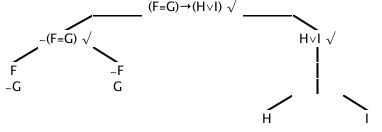#### Starting from a collection of formulas, rather than a single formula

Trees can be grown from a collection of formulas. Say we wished to draw a tree for F, (F→G), and ¬G, all of them at once together. One approach might be to think of them as being 'anded' together, and do a tree for the single composite formula F&(F→G)&~G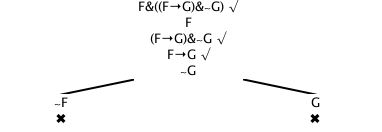But equally, and more simply, we could just write down all the members of the collection as the (extended) root and go on from there (instead of putting the 'ands' in then taking them out to get that point). Thus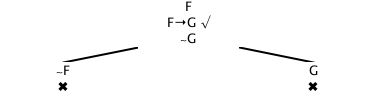[The last two trees are shown with their closed branches marked as closed by a cross.]

Starting from a collection or set of formulas will prove to be useful.

## Exercises to accompany Tree Tutorial 2

### Exercise 1 (of 1):

Try building some trees (click on the formula to select, choose 'extend' from the menu). Close branches if you can (click on a formula to select, click, or touch, on the negation of that formula (in the same branch), choose 'close' from menu).

Try building different trees from the same starting point (the order you apply the rules matters in terms of the tree generated) ie click on Tree 1, build it, click on Tree 1 again and build it again.

Notice

a) you cannot extend the same formula twice (once it is 'ticked' it is dead and unusable).
b) no rule applies to either an atomic formula or the negation of an atomic formula
c) you get a smaller tree if you delay using splitting rules (ie use 'straight ahead' rules first).
d) you get a smaller tree if you close branches as soon as you can.

If you decide to use the web application for the exercises you can launch it from here Deriver [default] — username 'logic' password 'logic'. Then either copy and paste

a) ~~A&(B∨C)&~~~C
b)  F&(F→G)&~G
c) ~((M↔N)∨(M↔~N))

into the Journal or use the Deriver File Menu to Open Web Page with this address https://softoption.us/test/treesStandAlone/CombinedTutorialsDefault.html . Then select the desired formula(s) and Start Tree off the Actions Menu.

### Preferences

[If you find you need to set the Preferences for the web application (because you, or someone else, has been doing something totally different with Deriver on the computer in use),

• set the parser to default

The title bar of the Deriver window will show you what system you are running e.g. 'DeriverDefault', 'DeriverGentzen', etc.]